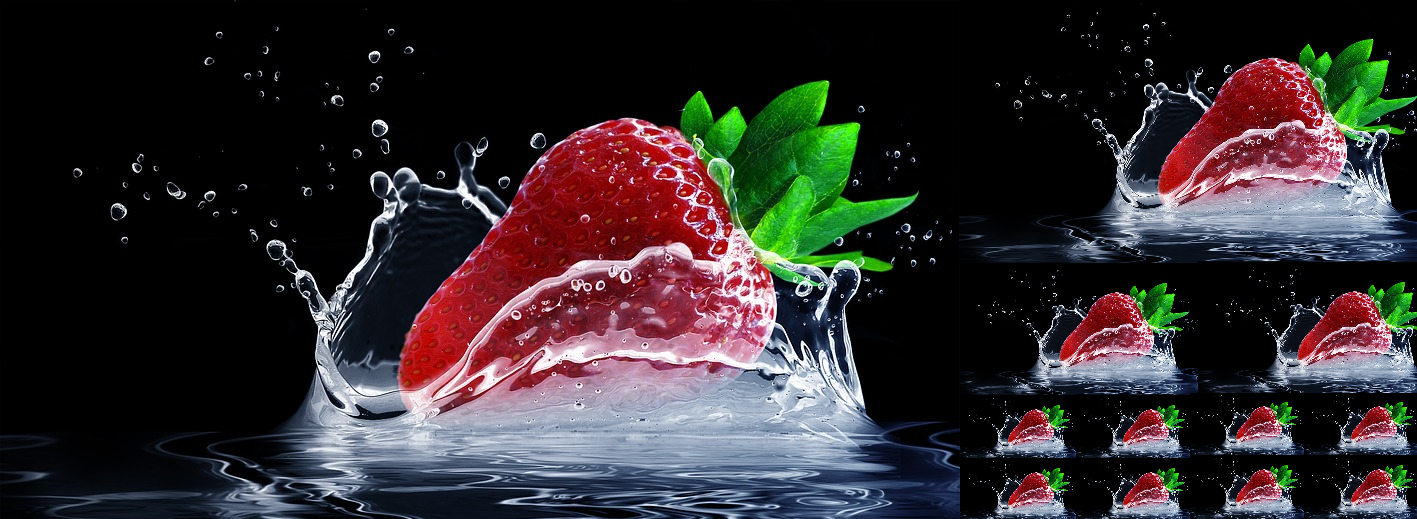In this post:

• pil imports
• resize an image and keep its aspect ratio 1
• resize an image and keep its aspect ratio 2
• Merge two images(a transparent with another one)
• Drawing Text
• Write mode P as JPEG
• Create a transparent image
• Convert RGBA to RGB image

You can check also : Python Frequently Used Scripts - strings, files and dates

## Frequently Used Scripts Intro:

This is a new series of Frequently Used Scripts FUS – little snippets and code examples that are designed to solve a particular problem. At some moment of completion of the series I’ll post them all together in a periodic table style. All of the scripts should be working alone only by copy and paste. It's relatively easy examples and you can start using it with little or even without any knowledge of programming.

## Python and PIL(python imaging)

Python is a very popular programming language with many practical areas like: artificial intelligence, automation, image manipulation, statistic and many other. Many people suggest that is perfect language to start learning programming with. Installation of python it's like installing any other application with some small changes for settings. Even some OS come with pre-installed python (like Linux mint).

PIL(python imaging) is image manipulation library which is very good for resizing, cropping, changing colors, merging or pasting images. It can be used also for animation and for "small" video editing.## pil imports

This imports are often used in pil scripts:

``````import os
import PIL
from PIL import Image
``````

## resize an image and keep its aspect ratio 1

By comparing the aspect ratios and considering the larger one, this will show what to cut off the sides or the top and bottom:

``````import Image

image = Image.open('images/sample.jpg')
width = image.size
height = image.size

if width > height:
difference = width - height
offset = difference / 2
resize = (offset, 0, width - offset, height)
else:
difference = height - width
offset = difference / 2
resize = (0, offset, width, height - offset)

thumb = image.crop(resize).resize((200, 200), Image.ANTIALIAS)
thumb.save('thumb.jpg')
``````

## resize an image and keep its aspect ratio 2

By comparing the aspect ratios and considering the larger one, this will show what to cut off the sides or the top and bottom:

``````import os, sys
import Image

size = 256, 256
for file in sys.argv[1:]:
outfile = os.path.splitext(file) + ".thumbnail"
if file != outfile:
try:
im = Image.open(file)
im.thumbnail(size, Image.ANTIALIAS)
im.save(outfile, "JPEG")
except IOError:
print "error creating thumbnail for '%s'" % file
``````
``````import PIL
from PIL import Image

basewidth = 400
img = Image.open('sample.jpg')
wpercent = (basewidth/float(img.size))
hsize = int((float(img.size)*float(wpercent)))
img = img.resize((basewidth,hsize), PIL.Image.ANTIALIAS)
img.save('output.jpg')
``````

## Merge two images(a transparent with another one)

Function paste() will be used:

• first parameter of .paste() is the image to paste.
• second are coordinates
• third parameter indicates a mask that will be used to paste the image.
``````import Image

background = Image.open("transperent.png")
foreground = Image.open("naormal.png")

background.paste(foreground, (0, 0), foreground)
background.show()
``````

## Combine two images (non transperent)

``````from PIL import Image

img = Image.open('/pathto/file', 'r')
img_w, img_h = img.size
background = Image.new('RGBA', (1440, 900), (255, 255, 255, 255))
bg_w, bg_h = background.size
offset = ((bg_w - img_w) / 2, (bg_h - img_h) / 2)

background.paste(img, offset)
background.save('out.png')
``````

## Drawing Text

If you need to "draw" a text message that fits exactly inside an image, then you can use the handy font.getsize method:

``````def draw_text(text, size, fill=None):
font = ImageFont.truetype(
'path/to/font.ttf', size
)
size = font.getsize(text)  # Returns the width and height of the given text, as a 2-tuple.
im = Image.new('RGBA', size, (0, 0, 0, 0))  # Create a blank image with the given size
draw = ImageDraw.Draw(im)
draw.text((0, 0), text, font=font, fill=fill)  # Draw text
return im
img = draw_text('Sample Text', 30, (82, 124, 178))
``````

## Write mode P as JPEG

If you get an error similar to:

"IOError: cannot write mode F as JPEG"

Then you can use this code in order to solve it:

``````Image.open('old.jpeg').convert('RGB').save('new.jpeg')
``````

## Create and paste image

Resize method should be used which a new image object. Then method pasted is used over the resized object:

`````` import Image

file = "sample.png"
img = Image.open(file)
resized = img.resize((75, 75))
r, g, b, alpha = resized.split()

newImage = Image.new(resized.mode, resized.size, "black")
newImage.save("out.png")
``````

## Create a transparent image

This script make a transparent image with a white circle drawn in the middle:

``````from PIL import Image, ImageDraw

img = Image.new('RGBA',(200, 200))
draw = ImageDraw.Draw(img)
draw.ellipse((25, 25, 75, 75), fill=(255, 255, 255))
img.save('circle.gif', 'GIF', transparency=0)
``````

## Convert RGBA to RGB image

Converting from RGBA to RGB using paste method by splitting and getting the alpha channel:

``````from PIL import Image
png = Image.open(object.logo.path)